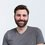# Just Some Game Theory

A famous artist is auctioning off his masterpiece, which everyone values at $2:There are two people who will bid, Alice and Bob. They have to put a whole dollar amount ($0, $1,$2, $3, ...) into an envelope (in secret). Both the winner and loser will have to pay whatever they put in the envelope; the highest bidder will get the painting, while the loser won't receive anything. If there is a tie, the painting is given to one of them at random. Assuming both people are trying to maximize their expected wealth, what would you if you were Alice?Note by Eli Ross 5 years, 4 months ago This discussion board is a place to discuss our Daily Challenges and the math and science related to those challenges. Explanations are more than just a solution — they should explain the steps and thinking strategies that you used to obtain the solution. Comments should further the discussion of math and science. When posting on Brilliant: • Use the emojis to react to an explanation, whether you're congratulating a job well done , or just really confused . • Ask specific questions about the challenge or the steps in somebody's explanation. Well-posed questions can add a lot to the discussion, but posting "I don't understand!" doesn't help anyone. • Try to contribute something new to the discussion, whether it is an extension, generalization or other idea related to the challenge. • Stay on topic — we're all here to learn more about math and science, not to hear about your favorite get-rich-quick scheme or current world events. MarkdownAppears as *italics* or _italics_ italics **bold** or __bold__ bold - bulleted- list • bulleted • list 1. numbered2. list 1. numbered 2. list Note: you must add a full line of space before and after lists for them to show up correctly paragraph 1paragraph 2 paragraph 1 paragraph 2 [example link](https://brilliant.org)example link > This is a quote This is a quote  # I indented these lines # 4 spaces, and now they show # up as a code block. print "hello world" # I indented these lines # 4 spaces, and now they show # up as a code block. print "hello world" MathAppears as Remember to wrap math in $$ ... $$ or $ ... $ to ensure proper formatting. 2 \times 3 $2 \times 3$ 2^{34} $2^{34}$ a_{i-1} $a_{i-1}$ \frac{2}{3} $\frac{2}{3}$ \sqrt{2} $\sqrt{2}$ \sum_{i=1}^3 $\sum_{i=1}^3$ \sin \theta $\sin \theta$ \boxed{123} $\boxed{123}$ ## Comments Sort by: Top Newest Suppose Alice and Bob put $A$ and $B$ dollars in the envelope respectively. If Alice wins, then her wealth would increase by $2 - A$ ( She gets a painting worth$2, but spends $$A$). If she loses, then her wealth would increase by $- A$. Note that if $A \geq 3$, then Alice's wealth would be decrease irrespective of whether she gets the painting or not since $2 - A < 0$. Therefore Alice should not put more than 2 dollars in the envelope. By symmetry, Bob should also not put more than 2 dollars. Putting$2 in the envelope is not a good idea either. If she wins, then her wealth remains the same. If she loses, then her wealth decreases. There is no positive outcome and there is risk of losing money, therefore Alice should not put $2 in the envelope. Again by symmetry, Bob should also not put$2.

We will now look at the remaining cases, that is $A, B \in \{ 0, 1 \}$, in the form of a matrix

$\begin{array}{|c|c|c|} \hline & 0 & 1 \\ \hline 0 & (2, 0) / (0, 2) = (1,1) & (0, 1) \\ \hline 1 & (1, 0) & (1, {-1}) / ({-1}, 1) = (0,0) \\ \hline \end{array}$

We see that putting $0 in the envelope is the dominant strategy for both players. It is also the only Nash equilibrium in this game. I feel this strategy is the best for Alice since she has a chance of getting$2, which is not possible in any other case. Also, there is no negative payoff; Alice does not risk losing any wealth even if she loses the game.

- 5 years, 4 months ago

If the players are risk-averse, then having 50/50 chance of getting $2 (if they both bid$0) is worse than having a sure $1 (if one player bids$1). Thus it becomes a coordination game, both of them trying to cooperate to bid different amounts.

- 5 years, 4 months ago

How will the players decide who pays $1 and who pays$0? Note that they are putting the amount in the envelope secretly. Will the players be willing to pay a dollar when they do not know what the other player is bidding?

- 5 years, 4 months ago

That's exactly the main discussion of cooperation game; look it up in Wikipedia.

- 5 years, 4 months ago

Since the game setting is symmetric, Alice and Bob will bid the same amount and this is a common knowledge to them.
Since they are trying to maximize their expected wealth, they will both bid \$0.

- 5 years, 4 months ago

Keep in mind they don't have to bid a fixed amount; for example, they could each choose to bid $\X$ with probability $P(X).$

Staff - 5 years, 4 months ago

So they will each bid at random according to their strategy with probability?

- 5 years, 4 months ago

In general, that's how all strategies work. You can think of a "fixed" strategy as a special case of this more general form, where $P(Y) = 1$ for some $Y.$

Staff - 5 years, 4 months ago

Oh I see...... I have only considered the "fixed" strategy...

- 5 years, 4 months ago

I liked this problem. Do we a wiki on game theory on Brilliant?

- 5 years, 4 months ago